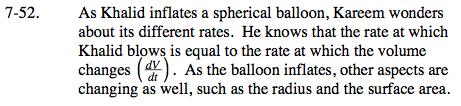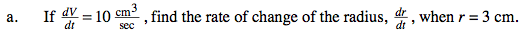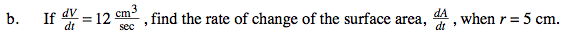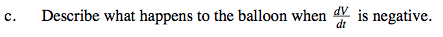### Home > CALC > Chapter Ch7 > Lesson 7.1.5 > Problem7-52

7-52.Using just geometry (no Calculus), find the equation for a generic spherical balloon.

$V=\frac{4}{3}\pi r^{3}$

Substitute the given information and solve.$\text{Find }\frac{dr}{dt}, \text{ you will need that information later.}$

Using just geometry, write the equation for the Surface Area of a generic spherical balloon: A = 4πr²What does a negative rate represent?